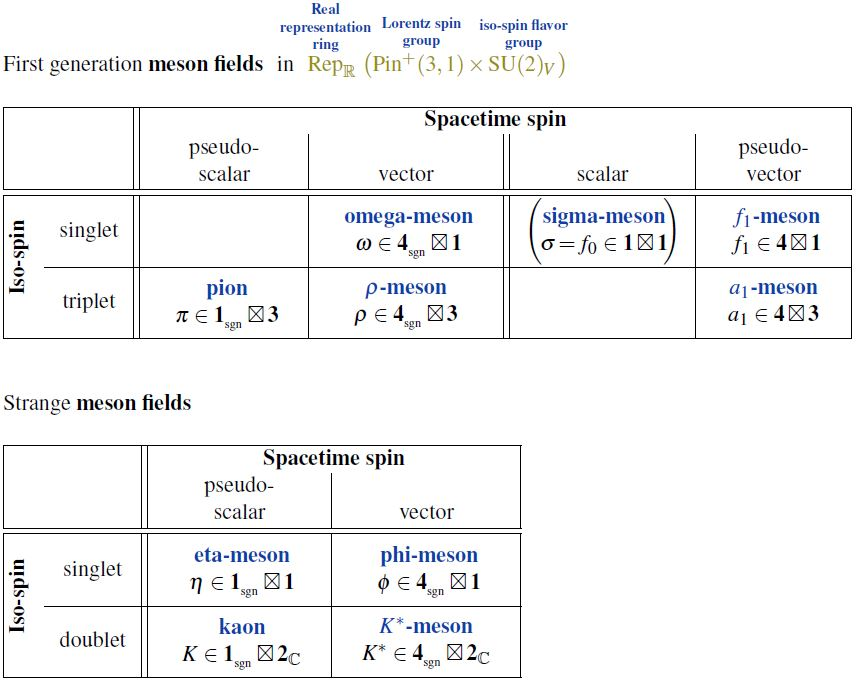# nLab f1-meson

Contents

### Context

#### Fields and quanta

fields and particles in particle physics

and in the standard model of particle physics:

force field gauge bosons

scalar bosons

flavors of fundamental fermions in the
standard model of particle physics:
generation of fermions1st generation2nd generation3d generation
quarks ($q$)
up-typeup quark ($u$)charm quark ($c$)top quark ($t$)
down-typedown quark ($d$)strange quark ($s$)bottom quark ($b$)
leptons
chargedelectronmuontauon
neutralelectron neutrinomuon neutrinotau neutrino
bound states:
mesonslight mesons:
pion ($u d$)
ρ-meson ($u d$)
ω-meson ($u d$)
f1-meson
a1-meson
strange-mesons:
ϕ-meson ($s \bar s$),
kaon, K*-meson ($u s$, $d s$)
eta-meson ($u u + d d + s s$)

charmed heavy mesons:
D-meson ($u c$, $d c$, $s c$)
J/ψ-meson ($c \bar c$)
bottom heavy mesons:
B-meson ($q b$)
ϒ-meson ($b \bar b$)
baryonsnucleons:
proton $(u u d)$
neutron $(u d d)$

(also: antiparticles)

effective particles

hadrons (bound states of the above quarks)

solitons

in grand unified theory

minimally extended supersymmetric standard model

superpartners

bosinos:

dark matter candidates

Exotica

auxiliary fields

# Contents

## Idea

The $f_1$-meson is the light meson which (in the Wigner classification) is the Lorentz spin group pseudo-vector representation and isospin scalar.

This is the chiral partner of the omega-meson.• Su Houng Lee, Observing chiral partners in nuclear medium (pdf)

• M. Kirchbach, D. O. Riska, The Coupling of the $f_1(1285)$ meson to the isoscalar axial current of the nucleon, Nucl. Phys.A 594 (1995) 419-424 (arXiv:nucl-th/9502018)

• Robert Pisarski, p. 1 of: Where does the Rho Go? Chirally Symmetric Vector Mesons in the Quark-Gluon Plasma, Phys. Rev. D52 (1995) 3773-3776 (arXiv:hep-ph/9503328)

• A. E. Dorokhova, N. I. Kochelevba. A. P. Martynenkoc. F. A. Martynenkoc. A. E. Radzhabovb, The contribution of axial-vector mesons to hyperfine structure of muonic hydrogen, Physics Letters B Volume 776, 10 January 2018, Pages 105-110 Physics Letters B (doi:10.1016/j.physletb.2017.11.027)